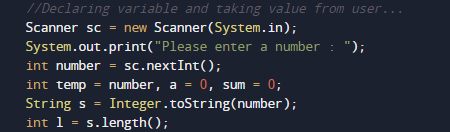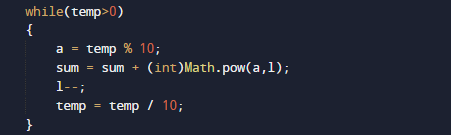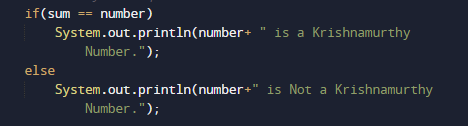# Program to check Krishnamurthy Number In Java

In this tutorial you will learn about the Program to check Krishnamurthy Number In Java and its application with practical example.

In this tutorial, we will learn to create a Java Program to check Krishnamurthy Numbers in Java using Java programming.

## Prerequisites

Before starting with this tutorial we assume that you are best aware of the following Java programming topics:

• Java Operators.
• Basic Input and Output function in Java.
• Class and Object in Java.
• Basic Java programming.
• If-else statements in Java.
• For loop in Java.

## What is  Krishnamurthy Number?

A number is called a to be Krishnamurthy if sum  of  its factorial digits is equal to that number is a Krishnamurthy number.

Example:suppose a number 145 then factors of 145 are => 5! + 4! + !1 = 1 + 24 + 120 = 145. So 145 is a Krishnamurthy.

## Program to check Krishnamurthy Number In Java

In this program we will find given number is a Krishnamurthy number or not in using java programming. We would first declared and initialized the required variables. Next, we would prompt user to input the a number.Later we  find number is Krishnamurthy number or not let’s have a look at the code.

## Output

Krishnamurthy NumberNot a Krishnamurthy Number.In the above program, we have first declared and initialized a set variables required in the program.

• number= it will hold entered number.
• temp= it will hold temporary original number.
• sum= it will hold sum of values.
• l= it will hold length of a number.And after that we calculate the value Sum of its digits powered with their respective position is equal to the original number or not using Math.pow(a,l). inbuilt function.So the given number called Krishnamurthy  if “sum of its digits powered with their respective position are equal” with the number.Number is Krishnamurthy if condition True if not then number is not a Krishnamurthynumber.

In this tutorial we have learn about the Program to check Krishnamurthy Number In Java and its application with practical example. I hope you will like this tutorial.